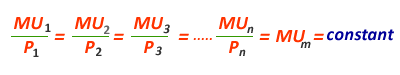# Equi-Marginal Principle

The Law of Equi-Marginal Utility is an extension to the law of diminishing marginal utility. The principle of equi-marginal utility explains the behavior of a consumer in distributing his limited income among various goods and services.

This law states that how a consumer allocates his money income between various goods so as to obtain maximum satisfaction.

Let us assume there are only three commodities available in the market, A, B and C. Also assume that Tom has a daily income of only \$15 to spend and that he can exactly order his utility preference for each of the three products. Product A costs \$1 per unit, Product B costs \$3 per unit and Product C costs \$5. Note that diminishing marginal utility sets in immediately for each of the three products. Marginal utility information is described on per \$ basis, because a consumers choice are influenced not only by the amount of additional utility that successive units give him but also how many dollars he give up to get them.

Let us consider each dollar spent. Marginal utility per dollar shows that one dollar spend on Product A provides the highest satisfaction of 20 utils as opposed to only 12 and 8 utils from products B and C, respectively.

Second dollar spends again buys the highest utility of 15 utils. However, when Tom spends the third dollar, a switch to Product B promises 15 utils of added satisfaction as opposed to 11 utils from Product A. Following the principle, the best combination Tom can purchase with \$15 would be 4 units of A, 2 units of B and 1 unit of C. The total utility generated would be 154 utils. \$4 spent on A give 54 utils of satisfaction; \$6 spent on Product B gives 60 utils and \$5 spent on C gives 40 utils. This gives a total of 154 utils. No other combination will result in as high utility as this with an expenditure of \$15.The results from the table above can be generalised to n commodities and the following condition should hold in equilibrium: